Next: Jack Spielberg - A Up: Operator Algebras / Algèbres Previous: Alexandru Nica - Some

## John Phillips - Spectral flow and index in bounded and unbounded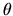-summable Fredholm modules: integral formulas

 JOHN PHILLIPS, Department of Mathematics and Statistics, University of Victoria, Victoria, British Columbia  V8W 3P4, Canada Spectral flow and index in bounded and unbounded-summable Fredholm modules: integral formulas

In joint work with Alan Carey, we study-summable Fredholm modules (H,D0) for Banach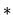-algebras, A, and integral formulas for the pairing of (H,D0) with K1(A). In particular, if (H, D0) is-summable (in Connes' original sense that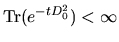for all t>0) then we prove that if u is a unitary in A1 with [u,D0] bounded and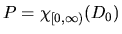, then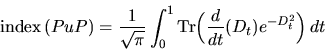where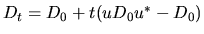is the straight line path from D0 to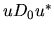. This is the pairing of [u] in K1(A) with [D0] in K1(A) and can also be interpreted as the spectral flow of the path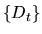. A proof of this formula was first outlined by Ezra Getzler.

Our proof is quite different from the one indicated by Getzler. Our method is able to handle Connes' new notion of-summability (which we dub weak--summability) i.e.,for some t>0. We can also handle the various bounded notions of-summability (indeed, our method is based on this). Finally our method simultaneously deals with the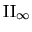-version of all these results.

Roughly speaking, our proofs involve three steps. First, given (H ,D0) we make a careful analytic study of the map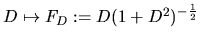defined for D in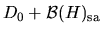. The pair (H,FD0) is a pre-Fredholm module for Awhich is-summable (in a restricted sense) and the FD vary in a certain affine space of bounded self-adjoint operators,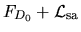. We show that this map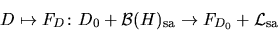is suitably smooth. Second, we study the various notions of bounded-summability that arise in this context. In particular, one of our results shows that if (H,F0) is a pre-Fredholm module which is-summable for A in the strongest sense, then for F in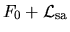and X in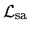(the tangent space toat F), Tr(X e-|1-F2|-1) is a closed (and exact) 1-form on the manifold,. The idea to use these differential-geometric notions in this context goes back to I.M. Singer and was also used by Getzler in his proof. The point here is that now the integral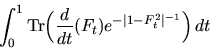is seen to be independent of path in the space,. Third, we can now reduce to the case of genuine (bounded,-summable) Fredholm modules (H,F0) (i.e., F02=1), and paths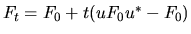. In this setting, P=2F0-1and the formula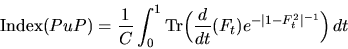is still not a triviality.

As mentioned above, our results are proved in such a way that the typecase is included at all stages.Next: Jack Spielberg - A Up: Operator Algebras / Algèbres Previous: Alexandru Nica - Some